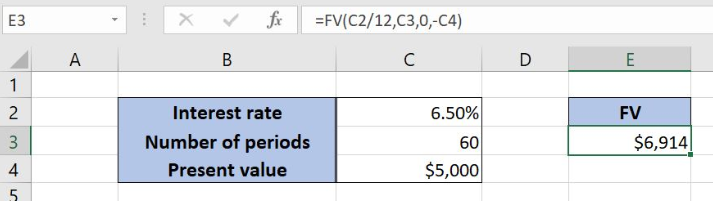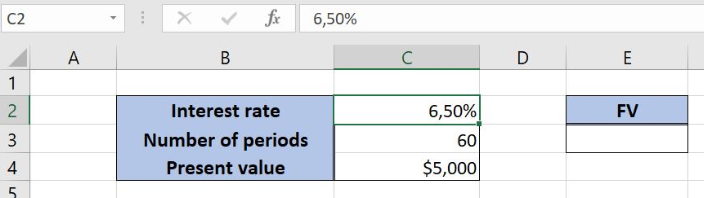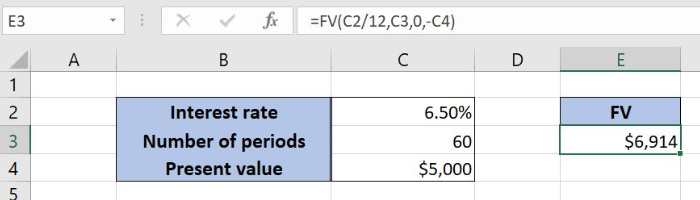Get instant live expert help with Excel or Google Sheets“My Excelchat expert helped me in less than 20 minutes, saving me what would have been 5 hours of work!”

#### Post your problem and you’ll get Expert help in seconds.

Your message must be at least 40 characters
Our professional Expert are available now. Your privacy is guaranteed.

# Calculate COMPOUND Interest

Excel allows a user to calculate the compound interest of an investment using the FV function. This step by step tutorial will assist all levels of Excel users in calculating the compound interest of an investment.Figure 1. The result of the FV function

## Syntax of the FV Formula

The generic formula for the FV function is:

`=FV (rate, nper, pmt, [pv], [type])`

The parameters of the FV function are:

• rate – an interest rate for the period (the annual rate divided by 12)
• nper – the total number of periods of an investment
• pmt – payment per period
• [pv] – the present value of a loan and must be negative. This is an optional parameter. If it’s omitted, the default value is 0
• [type] –  specifies when the payments are made: at the end of a period (0) or at the beginning of a period (1). By default, it’s 0.

## Setting up Our Data for the FV Function

Let’s look at the structure of the data we will use. In the cell C2, we have the annual interest rate for the loan. In the cell C3 is the number of periods for the investment. In the C4 is the present value of the loan. We want to get the result of the FV function in the cell E3.Figure 2. Data that we will use in the FV example

## Calculate the Compound Interest of the Investment

In our example, we want to calculate the compound interest of the investment in the cell E3. The interest rate is 6.50%, the total number of periods is 60 and the present value of the investment is \$5,000.

The formula looks like:

`=FV(C2/12, C3, 0, -C4)`

The parameter rate is C2/12, as we must pass the monthly interest rate to the function. The nper parameter is C3 (60) and the pmt is 0. The pv is in the C4 (\$5,000), with the minus.

To apply the FV function, we need to follow these steps:

• Select cell E3 and click on it
• Insert the formula: `=FV(C2/12, C3, 0, -C4)`
• Press enterFigure 3. Using the FV function to calculate the compound interest of the investment

Finally, the result in the cell E3 is \$6,914, which is the compound interest of the investment of \$5,000 in 60 months with the annual interest rate of 6,50%.

Most of the time, the problem you will need to solve will be more complex than a simple application of a formula or function. If you want to save hours of research and frustration, try our live Excelchat service! Our Excel Experts are available 24/7 to answer any Excel question you may have. We guarantee a connection within 30 seconds and a customized solution within 20 minutes.